Class X Math
HOTS for Arithmetic Progressions
1.    Find the ‘6th’ term of the A.P.: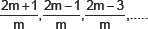2.    If the numbers a, b, c, d and e form an A.P., then find the value of a – 4b + 6c – 4d + e.
3.    If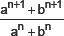is the arithmetic mean between ‘a’ and ‘b’, then, find the value of ‘n’.
4.    If pth term of an A.P. is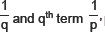prove that the sum of the first ‘pq’ terms is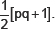5.    If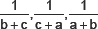are in A.P., prove that a2, b2, c2 are also in A.P.
6.    Solve the equation:
1 + 4 + 7 + 10 + ... + x = 287
7.    Find three numbers in A.P. whose sum is 21 and their product is 231.
8.    Find p and q such that: 2p, 2p, q, p + 4q, 35 are in AP
9.    If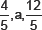are three consecutive terms of an AP, find the value of a.
10.    For what value of p, are (2p – 1), 7 and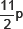three consecutive terms of an AP?